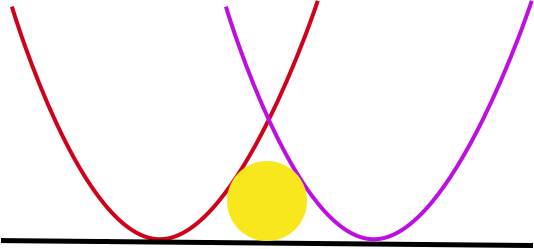# No way out

Calculus Level 5Let $S$ be the region in the first quadrant which is

(i) to the right of the parabola $y = x^{2}$ and

(ii) to the left of the parabola $y = (x - 2)^{2}$.

Let $r$ be the radius of the largest circle which can be inscribed in $S$. Find $\lfloor 10000*r \rfloor$.

×